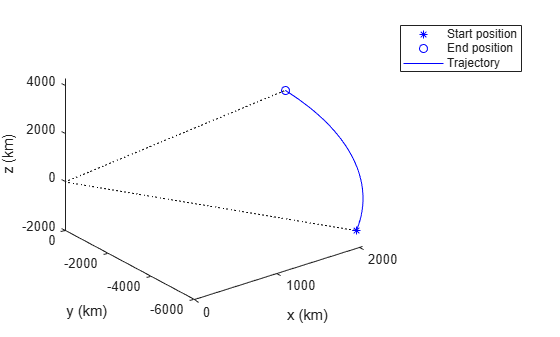# Platform.pose

Pose of platform

Since R2018b

## Syntax

``pse = pose(ptfm)``
``pse = pose(ptfm,type)``
``pse = pose(___,'CoordinateSystem',coordinate)``

## Description

````pse = pose(ptfm)` returns the estimated pose, `pse`, of the platform `ptfm`, in scenario coordinates. The platform must already exist in the tracking scenario. Add platforms to a scenario using the `platform` method. The pose is estimated by a pose estimator specified in the `PoseEstimator` property of the platform.```
````pse = pose(ptfm,type)` specifies the type of the pose estimation as `'estimated'` or `'true'`.```

example

````pse = pose(___,'CoordinateSystem',coordinate)` specifies the coordinate system of the `pse` output. You can only use this syntax when the `IsEarthCentered` property of the tracking scenario is set to `true`.```

## Examples

collapse all

Create a tracking scenario with a specified update rate.

`scene = trackingScenario('IsEarthCentered',true,'UpdateRate',0.01);`

Add an airplane in the scenario. The trajectory of the airplane changes in latitude and altitude.

`plane = platform(scene,'Trajectory',geoTrajectory([-12.338,-71.349,10600;42.390,-71.349,0],[0 36000]));`

Advance the tracking scenario and record the geodetic and Cartesian positions of the plane target.

```positions = []; while advance(scene) poseLLA = pose(plane,'CoordinateSystem','Geodetic'); poseCart = pose(plane,'CoordinateSystem','Cartesian'); positions = [positions;poseCart.Position];%#ok<AGROW> Allow the buffer to grow. end```

Visualize the trajectory in the ECEF frame.

```figure() km = 1000; % Plot the trajectory. plot3(positions(1,1)/km,positions(1,2)/km,positions(1,3)/km, 'b*'); hold on; plot3(positions(end,1)/km,positions(end,2)/km,positions(end,3)/km, 'bo'); plot3(positions(:,1)/km,positions(:,2)/km,positions(:,3)/km,'b'); % Plot the Earth radial lines. plot3([0 positions(1,1)]/km,[0 positions(1,2)]/km,[0 positions(1,3)]/km,'k:'); plot3([0 positions(end,1)]/km,[0 positions(end,2)]/km,[0 positions(end,3)]/km,'k:'); xlabel('x (km)'); ylabel('y (km)'); zlabel('z (km)'); legend('Start position','End position','Trajectory')```## Input Arguments

collapse all

Scenario platform, specified as a `Platform` object. To create platforms, use the `platform` method.

Source of platform pose information, specified as `'estimated'` or `'true'`. When set to `'estimated'`, the pose is estimated using the pose estimator specified in the `PoseEstimator` property of the tracking scenario. When `'true'` is selected, the true pose of the platform is returned.

Example: `'true'`

Data Types: `char`

Coordinate system to report pose, specified as:

• `'Cartesian'` — Report poses using Cartesian coordinates in the Earth-Centered-Earth-Fixed coordinate frame.

• `'Geodetic'` — Report positions using geodetic coordinates (latitude, longitude, and altitude). Report orientation, velocity, and acceleration in the local reference frame (North-East-Down by default) corresponding to the current waypoint.

.

You can only use this argument when the `IsEarthCentered` property of the tracking scenario is set to `true`.

## Output Arguments

collapse all

Pose of platform, returned as a structure. Pose consists of the position, velocity, orientation, and angular velocity of the platform with respect to scenario coordinates. The returned structure has these fields:

FieldDescription
`PlatformID`

Unique identifier for the platform, specified as a positive integer. This is a required field with no default value.

`ClassID`

User-defined integer used to classify the type of target, specified as a nonnegative integer. Zero is reserved for unclassified platform types and is the default value.

`Position`

Position of platform in scenario coordinates, specified as a real-valued 1-by-3 row vector.

• If the `coordinateSystem` argument is specified as `'Cartesian'`, the `Position` is the 3-element Cartesian position coordinates in meters.

• If the `coordinateSystem` argument is specified as `'Geodetic'`, the `Position` is the 3-element geodetic coordinates: latitude in degrees, longitude in degrees, and altitude in meters.

`Velocity`

Velocity of platform in scenario coordinates, specified as a real-valued 1-by-3 row vector. Units are meters per second. The default value is ```[0 0 0]```.

`Acceleration`

Acceleration of the platform in scenario coordinates, specified as a 1-by-3 row vector in meters per second squared. The default value is `[0 0 0]`.

`Orientation`

Orientation of the platform with respect to the local scenario navigation frame, specified as a scalar quaternion or a 3-by-3 rotation matrix. Orientation defines the frame rotation from the local navigation coordinate system to the current platform body coordinate system. Units are dimensionless. The default value is `quaternion(1,0,0,0)` or a 3-by-3 identity matrix.

`AngularVelocity`

Angular velocity of the platform in scenario coordinates, specified as a real-valued 1-by-3 vector. The magnitude of the vector defines the angular speed. The direction defines the axis of clockwise rotation. Units are degrees per second. The default value is `[0 0 0]`.

## Version History

Introduced in R2018b